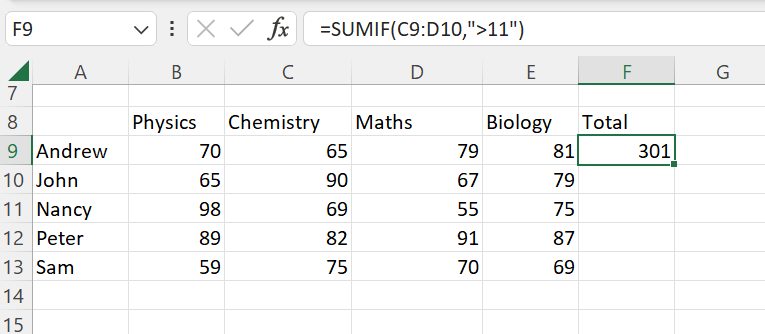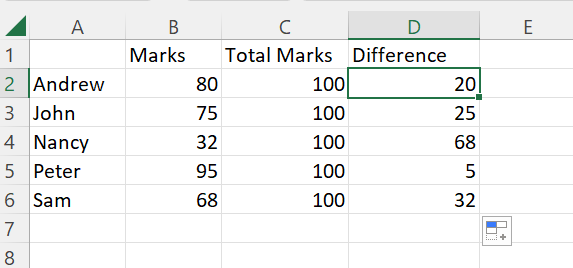top of page
Search

# Excel Formulas and Excel Shortcuts

Updated: Jan 26

In this article, we will explain to you the most used Microsoft Excel Formulas and Shortcuts.Why we need Excel Formulas:

Excel is necessary for everyone working in any industry that requires tabular data. Knowing how to use Excel will make your life much more straightforward, whether in business, as a professional, or as a teacher.

1. Helpful in organizing client sales lists, budgeting, and stock analysis in the financial sector.

2. Useful for e-commerce enterprises to spot patterns and classify data into meaningful groups.

3. Carries out human resources tasks like classifying hours worked and arranging employee profiles and expenses.

4. Its other applications include content calendars, consumer data collection, invoicing, and inventory tracking and administration.

5. Its ability to quickly integrate with other data pipelines and automation tools makes it unique. For instance, Zapier enables you to link thousands of popular apps with Microsoft Excel.

## Microsoft Excel Formulas

Microsoft Excel Formula performs calculations, mathematical operations, and other data processing tasks. It also allows you to work with date and time variables and do numerous other operations like finding averages and percentages for a group of cells.

Here we have most used Microsoft Excel Formulas:

1. Average

2. Array

3. Count

4. Division

5. Date

6. Multiplication

7. Sum

8. SumIf

9. if

10. Subtraction

11. Percentage

12. Randomize

13. Trim

14. Vlookup

15. Left, Mid, and Right

### Average

It is used to compute the data's average. The average is provided in a row or column by adding all numeric values and dividing the result by the number of numeric values.

1. Create a table.

2. Go to Editing and select Average.

or simply you can write the formula =AVERAGE(B2:B6). (See the image below)### Array

It is used to carry out complicated computations that are frequently impossible to do manually. The two basic categories of the ARRAY Formula are:

1. Return Array of values

2. Return Single value### Count

It is used to count the number of cells in a given array or how many cells are present in a specific range of numbers.

The syntax is =COUNT (D4:D8)### Division

It is used to divide the values. But there is no specific formula for Division. You have to use "/" to use the division function.

For example, =D/10 is used to divide values from column D by 10.### Date

It is used to combine specific years, months, and days from other cells to construct dates. It can also be used to alter the dates in Excel Worksheet.

The syntax is =DATE (year, month, day).You can change the format of the date.

Right-click on the date (D10) and select "Format cells".

Then go to date and select the format you want. Click OK.### Multiplication

It is used to multiply numbers using the asterisk(*) symbol.

The syntax is =column * column (C4*D4)

If you want to multiply specific number of columns then use =PRODUCT (C4, C6, C8)### Sum

the SUM function is used to combine several numbers, add a single number to a range of numbers, add numbers from one column to another, etc. In simple words, it is used to add numbers from alternate cells.

The syntax is =C6+C8+ C10+C12### SumIf

The SUMIF function is used to add numbers that meet specific conditions. For example, if you want to add numbers greater than 11 from a range of numbers, the SUMIF function is used.

You can also use SUMIF to add numbers depending on a Text condition. It is a very flexible formula that you may use to integrate, based on circumstances, into any Excel page.

The syntax is =SUMIF(C4:C10, ">11")### if

It is used to enable logical comparisons between values and output returns Text and values both can be evaluated using the IF function. The IF function performs a logical test and returns a value or text for both a TRUE and a FALSE outcome.

The syntax is =IF(logical test, value if true, value if false).### Subtraction

It is used to subtract the values from one column to another column. The subtraction formula will be duplicated in all cells if you drag the corner of the D6 cell.

The syntax is =C4-D4### Percentage

It is used to determine the percentage of various data including exam correct answers the percentage, or discount. The percentage is calculated as the proportion per hundred in mathematical procedures. In this sense, the denominator and numerator are divided, and the resulting number is multiplied by 100.### Trim

It is used to eliminate extra leading or trailing space. When formatting a giant Excel sheet, the TRIM function is helpful because all you have to do is specify the cell range to have any extra spaces removed.

The syntax is =TRIM (column) or =TRIM (C9)### Vlookup

It is used to find something in a large table or range of cells. It is used to locate an item's number, text, etc., inside a particular range.

The syntax is =VLOOKUP (G5, B4:E9, 4, False)

• G5: This cell contains the search term or number that you want to locate from the large table or workbook

• B4:E9: This is the table range wherein VLOOKUP searches.

• 4: Instructs the VLOOKUP function to return the value from the fourth column of the table in the same row.

• FALSE: It returns an error if the match is not found.### Left, Mid, and Right

It is used to extract a specific character, word, or number from an Excel cell.

The Syntax is =LEFT (text, num)## Microsoft Excel Shortcuts:

Microsoft Excel Shortcut allows you to complete tasks fast and more efficiently without going through the menu.

Below we have some useful Excel Shortcuts to simplify your data efficiently:

Ctrl + Shift + 1 [!] - To apply the standard number format.

Ctrl + N - To start a new worksheet

Ctrl + 0 - To open the existing worksheet

Ctrl + S - To save the worksheet

Ctrl + W - To close the worksheet

Ctrl + PageDown - Take you to the next sheet

Ctrl + PageUp - Take you to the previous sheet.

Alt + A - Take you to the Data Tab.

Alt + W - Take you to the View Tab.

Alt + M - Bring up the Formula Tab.

Ctrl + ; - To add the current date.

Ctrl + Shift + ; - To insert the current date.

Ctrl + R - Copy formulas to adjacent column cells

Ctrl + D - Copy formulas to the next row cell.

Shift + Down Arrow key - Select multiple cells

Ctrl + C - Copy several cells

Ctrl + V - Paste numerous cells

F5 + Ctrl + G - go back to previous locations

Ctrl + C (to copy), after that Shift + F10 + V - To change the formula to value

Ctrl + Space - To select the entire row or column

The Tech Platform